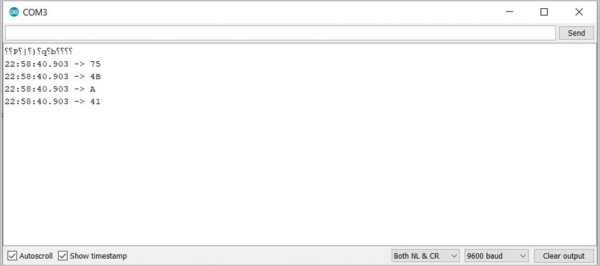# Print hexadecimal values in Arduino

In order to print hexadecimal equivalents of numbers or characters, adding 'HEX' as the second argument of Serial.print() will be sufficient.

The following code demonstrates this −

## Example

void setup() {
// put your setup code here, to run once:
Serial.begin(9600);
Serial.println();
Serial.println(75);
Serial.println(75, HEX);
Serial.println('A');
Serial.println('A',HEX);
}
void loop() {
// put your main code here, to run repeatedly:

}

The corresponding Serial monitor output is −Now, the conversion of the decimal number 75 to a hexadecimal value is straightforward and you can even verify that 0x4B is the correct hexadecimal representation of 75. But what does the hexadecimal representation of 'A' mean?

Well, it is the hexadecimal representation of the number corresponding to A in the ASCII system. You can have a look at the ASCII table here: https://www.cs.cmu.edu/~pattis/15-1XX/common/handouts/ascii.html

As you can see, 'A' corresponds to number 65, whose hex representation would be 41.

Please note that hex representations do not work for floating point numbers. Serial.println(1.912,HEX); will only print 1.912.